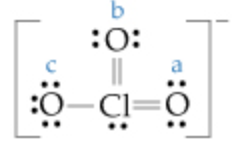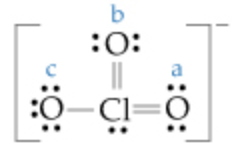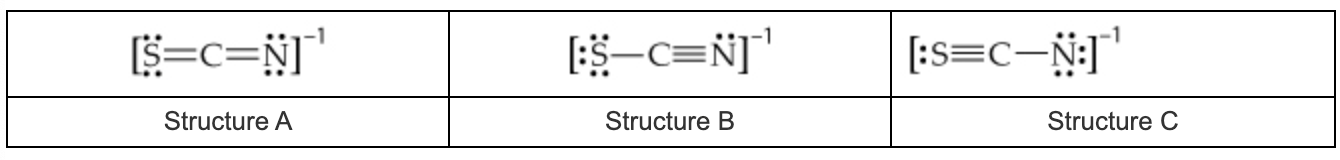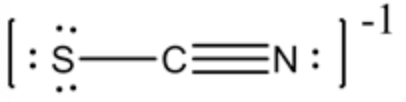In: Chemistry

# The Lewis structure for the chlorate ion is Calculate the formal charge on the chlorine (Cl) atom

A) The Lewis structure for the chlorate ion isCalculate the formal charge on the chlorine (Cl) atom.

B)

Calculate the formal charge on each of the oxygen (O) atoms labeled $$\mathrm{a}, \mathrm{b},$$ and $$\mathrm{c}$$ in the following Lewis structure.C)

What are the formal charges on the sulfur (S), carbon (C), and nitrogen (N) atoms, respectively, in the resonance structure that contributes most to the stability of the thiocyanate ion, SCN\%u2212?

The possible resonance structures for the thiocyanate ion, SCN\%u2212, are## Solutions

##### Expert Solution

Concepts and reason

A Lewis structure is a diagram that represents the chemical bonding between atoms of molecules and a lone pair of electrons that may exist in the molecule. It is also called a dot structure representing a lone pair and bond pair of electrons in the molecule. Lewis structure is used to draw covalently bonded molecules as well as coordination compounds.

Fundamentals

- Lewis's structure represents the bonding and lone pair of electrons in the molecule.

- Valence electrons are the electrons present in the outermost shell of an atom. Dots represent the electron position around the atoms, and lines or dot pairs are used to represent covalent bonds between atoms.

- The Lewis structure is based on the concept of the octet rule. So, the electrons shared in each atom should have 8 electrons in its outer shell.

- The formal charge (F.C) of the atom can be calculated by using the following formula.

$$\left(\begin{array}{l} \text { Formal } \\ \text { charge (F.C) } \end{array}\right)=\left(\begin{array}{l} \text { no.ofvalence } \\ \text { electronsinatom } \end{array}\right)-\frac{1}{2}\left[\begin{array}{l} \text { no.ofbonding } \\ \text { electrons } \end{array}\right] -\left[\begin{array}{l}\text { no.ofnon }-\text { bonding } \\ \text { electrons }\end{array}\right]$$

Part A

The formal charge on the chlorine atom in the chlorate ion is zero.

Number of valance electron for Clis7

Number of bonding electrons for Clis10

Number of non - Bonding electrons for Clis2

Substituting these numbers in formal charge (FC)

$$\mathrm{FC}=7-\left(\frac{1}{2} \times 10\right)-2$$

$$=0$$

Therefore, the formal charge on the chlorine atom is zero in the chlorate ion.

Part B

Formal charge on oxygen $$(\mathrm{a})=0$$

Formal charge on oxygen $$(\mathrm{b})=0$$

Formal charge on oxygen $$(\mathrm{c})=-1$$

Part B The charge on each oxygen atom is $$0,0,-1 .$$

Oxygen(a) :

Number of valance electron for $$\mathrm{O}(\mathrm{a})=6$$

Number of bonding electrons for $$\mathrm{O}(\mathrm{a})=4$$

Number of non $$-$$ Bonding electrons for $$\mathrm{O}(\mathrm{a})=4$$

Substituting these numbers in formal charge :

$$\mathrm{FC}=6-\left(\frac{1}{2} \times 4\right)-4$$

$$=0$$

Oxygen(b) :

Number of valance electron for $$\mathrm{O}(\mathrm{b})=6$$

Number of bonding electrons for $$\mathrm{O}(\mathrm{b})=4$$

Number of non $$-$$ Bonding electrons for $$\mathrm{O}(\mathrm{b})=4$$

Substituting these numbers in formal charge :

$$\mathrm{FC}=6-\left(\frac{1}{2} \times 4\right)-4$$

$$=0$$

Oxygen(c)

Number of valance electron for $$\mathrm{O}(\mathrm{c})=6$$

Number of bonding electrons for $$\mathrm{O}(\mathrm{c})=2$$

Number of non $$-$$ Bonding electrons for $$\mathrm{O}(\mathrm{c})=6$$

Substituting these numbers in formal charge :

$$\mathrm{FC}=6-\left(\frac{1}{2} \times 2\right)-6$$

$$=-1$$

Therefore, the formal charge on each oxygen atom is $$0,0,-1 .$$

Part C

The most stable structure is structure $$B$$ :For the structure B:

Formal charge on sulfur(S) $$=0$$

Formal charge on carbon $$(\mathrm{C})=0$$

Formal charge on nitrogen $$(\mathrm{N})=-1$$

Part C The formal charge on sulfur, carbon and nitrogen is 0,0,-1 .

Sulfur(S) :

Number of valance electron for $$\mathrm{S}=6$$

Number of bonding electrons for $$\mathrm{S}=4$$

Number of non - Bonding electrons for $$\mathrm{S}=4$$

Substituting these numbers in formal charge :

$$\mathrm{FC}=6-\left(\frac{1}{2} \times 4\right)-4$$

$$=0$$

$$\operatorname{Carbon}(\mathrm{C})$$

Number of valance electron for $$\mathrm{C}=4$$

Number of bonding electrons for $$\mathrm{C}=8$$

Number of non $$-$$ Bonding electrons for $$\mathrm{C}=0$$

Substituting these numbers in formal charge :

$$\mathrm{FC}=4-\left(\frac{1}{2} \times 8\right)-0$$

$$=0$$

Nitrogen $$(\mathrm{N}):$$

Number of valance electron for $$\mathrm{N}=5$$

Number of bonding electrons for $$\mathrm{N}=4$$

Number of non $$-$$ Bonding electrons for $$\mathrm{N}=4$$

Substituting these numbers in formal charge :

$$\mathrm{FC}=5-\left(\frac{1}{2} \times 4\right)-4$$

$$=-1$$

Therefore, the formal charge on sulfur, carbon and nitrogen is 0,0,-1